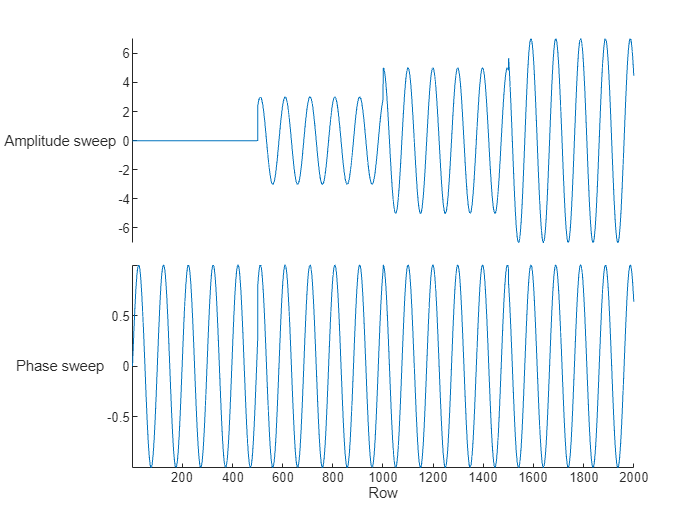# Read Multiple TDMS-Files into MATLAB

This example shows how to use a TDMS datastore to read data from multiple TDMS-files into MATLAB® for analysis.

The multiple TDMS-files of this example contain measurement data of a sine wave amplitude and phase, based on a trigger.

#### Read Multiple TDMS-Files at Once

The `tdmsDatastore` function creates a datastore object, which allows you to treat all the TDMS-files in a folder as one dataset.

```folderName = fullfile(pwd,"Trigger"); ds = tdmsDatastore(folderName);```

Review all the channels in the dataset.

`ds.ChannelList`
```ans=2×8 table ChannelGroupNumber ChannelGroupName ChannelGroupDescription ChannelName ChannelDescription Unit DataType NumSamples __________________ ________________ _______________________ _________________ __________________ ____ ________ __________ 1 "Trigger" "" "Amplitude sweep" "" "" "Double" 500 1 "Trigger" "" "Phase sweep" "" "" "Double" 500 ```

All the TDMS-files in the datastore must have the same channel groups and channels. The NumSamples in the channel list is that of the first file in the datastore.

The `readall` function retrieves all the data from the datastore into MATLAB. Read and plot the data from the first channel group.

```data = readall(ds); stackedplot(data{1})```You can also analyze data in the datastore one file at a time. Redefine the TDMS datastore with a read size of `"file"`.

`ds = tdmsDatastore(folderName, readSize="file");`

Iterate through the datastore, reading data from each TDMS-file. The `read` function uses the set read size. For each file, find its maximum and minimum values in the `Amplitude sweep` channel, and compare it to the cumulative maximum and minimum.

```maxAmplitude = 0; minAmplitude = 0; while(hasdata(ds)) data = read(ds); maxAmplitude = max(maxAmplitude, max(data{1}.("Amplitude sweep"))); minAmplitude = min(minAmplitude, min(data{1}.("Amplitude sweep"))); end```

After the entire dataset is read, view the cumulative maximum and minimum amplitudes.

`maxAmplitude`
```maxAmplitude = 6.9998 ```
`minAmplitude`
```minAmplitude = -6.9982 ```